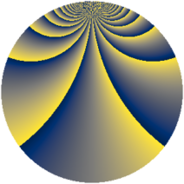# Properties

 Label 1104.2.bkLevel $1104$ Weight $2$ Character orbit 1104.bk Rep. character $\chi_{1104}(95,\cdot)$ Character field $\Q(\zeta_{22})$ Dimension $480$ Sturm bound $384$

# Related objects

## Defining parameters

 Level: $$N$$ $$=$$ $$1104 = 2^{4} \cdot 3 \cdot 23$$ Weight: $$k$$ $$=$$ $$2$$ Character orbit: $$[\chi]$$ $$=$$ 1104.bk (of order $$22$$ and degree $$10$$) Character conductor: $$\operatorname{cond}(\chi)$$ $$=$$ $$276$$ Character field: $$\Q(\zeta_{22})$$ Sturm bound: $$384$$

## Dimensions

The following table gives the dimensions of various subspaces of $$M_{2}(1104, [\chi])$$.

Total New Old
Modular forms 2040 480 1560
Cusp forms 1800 480 1320
Eisenstein series 240 0 240

## Trace form

 $$480q + O(q^{10})$$ $$480q - 24q^{21} + 48q^{25} + 48q^{49} + 48q^{57} + 240q^{81} + 48q^{85} + 72q^{93} + O(q^{100})$$

## Decomposition of $$S_{2}^{\mathrm{new}}(1104, [\chi])$$ into newform subspaces

The newforms in this space have not yet been added to the LMFDB.

## Decomposition of $$S_{2}^{\mathrm{old}}(1104, [\chi])$$ into lower level spaces

$$S_{2}^{\mathrm{old}}(1104, [\chi]) \cong$$ $$S_{2}^{\mathrm{new}}(276, [\chi])$$$$^{\oplus 3}$$